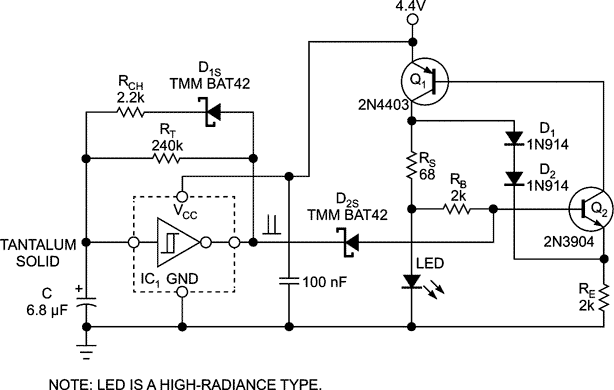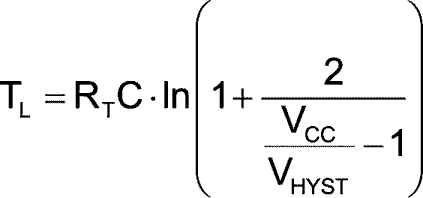# Low-duty-cycle LED flasher keeps power draw at 4 mW

Marian Stofka

EDN

Battery-operated equipment often will benefit from a power-on indicator. The indicator, however, can waste significant power. In situations where a low-duty-cycle blinking indicator provides an adequate indication of the power being turned on, the simple circuit described here should prove useful.Figure 1. Q1 and Q2 function as a current source and push a constant current through the LED regardless of its forward voltage drop (within the compliant voltage limitations). The Schmitt inverter forms a classic square-wave generator, modified with RCH and D1S to produce an asymmetrical output.

A tiny, single-gate Schmitt-trigger logic inverter, the SN74AHC1G14, together with two resistors, a Schottky diode, and a capacitor form the timing generator of the blinker, shown in Figure 1. The output waveform has a period of about 0.5 sec and a very low duty-cycle value, of around 1%. The interval of low-output duration, TL, of the generator is expressed aswhere

VHYST is the hysteresis voltage at the input of IC1,
VCC is the supply voltage of IC1.

For VCC=4.5V, the typical value for VHYST is 0.75V. For the required value of TL=0.5 sec, a value for RT of 200k was selected. The value of the timing capacitor, C, can be calculated from the equation, with a small amount of algebraic rearranging, as 7.45 μF. The nearest standard value is 6.8 μF; a tantalum solid-electrolytic capacitor is used for this value. To achieve the low duty cycle of the generator, the high-output duration, TH, is shortened by speeding up the time to charge capacitor C. This is done through the additional resistor, RCH, and the series-connected Schottky diode, D1S. The forward voltage drop at D1S is no more than 200 mV and can be neglected. The LED is on for approximately (1/100) × TL ≈ 5 msec.

The LED driver comprises a PNP bipolar transistor, Q1, and an NPN bipolar transistor, Q2. Q1 and Q2 form a switchable current source. At a high logic level at the cathode of Schottky diode D2S, a constant current flows through the LED with a value of roughly IO ≈ 0.7V/RS, or about 10 mA in this circuit.

Series-connected silicon diodes D1 and D2 provide strong nonlinear negative feedback. If for any reason the voltage drop across the sensing resistor, RS, rises, the D1-to-D2 connection will force almost the same increase in voltage at the emitter of Q2. This increase reduces the collector current of Q2 and, therefore, the base current of Q1 and closes the loop; the net result is a reduction of the collector current of Q1 to maintain a constant value.

Note that when the output of IC1 goes low, the current through D2S and resistor RB is negligible. This is due to the fact that, with the output of IC1 low, the base of Q2 is held low, turning it and the current source off. With the current source off, the LED is off as well, and only microamps of leakage current flow through D2S and RB. If you use all surface-mount devices, you can build the circuit on a board no larger than 16×16 mm.

This work was supported by the Slovak Research and Development Agency under contract no. APVV-0062-11.

EDN